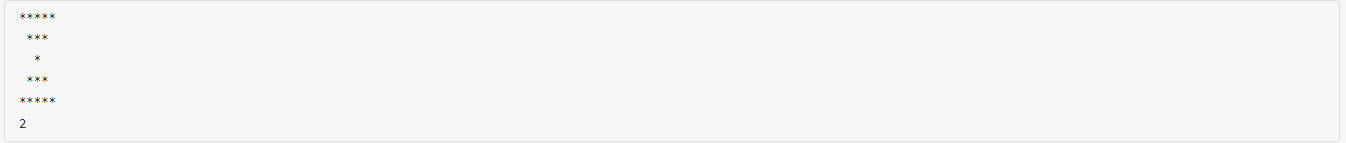## L1-002 打印沙漏 (20分)（易错点分析+代码）19 *``````#include <iostream>
#include <math.h>
using namespace std;
int main()
{
int n;
char ch;
cin>>n>>ch;
int r=0,sum=0,count=0;
if(n==1)
cout<<ch<<endl<<0<<endl;
else
{
for(int i=0;;i++){
if(sum>=n)
break;
sum+=(i*2+1)*2-1;
r=i;
}
int r_sum=(r-1)*2+1;
for(int i=0;i<r;i++){
if(i==r-1)
break;
for(int a=0;a<((r-1)*2+1-r_sum)/2;a++)
cout<<" ";
for(int j=0;j<r_sum;j++){
count++;
cout<<ch;
}
r_sum-=2;
cout<<endl;
}
int flag=1;
for(int i=0;i<r;i++){
for(int k=0;k<((r-1)*2+1-flag)/2;k++){
cout<<" ";
}
for(int j=0;j<flag;j++){
cout<<ch;
count++;
}
flag+=2;
cout<<endl;
}
cout<<n-count<<endl;
}
return 0;
}
``````